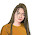## Thursday, September 3, 2020

### MATHS PARI ANDROID APP

MATHS PARI ANDROID APP

• Maths Pari is an app for online study from standard 1 to 10. developed by baldev pari and janardan pari.
• Treasure in a single applicationStunning application created by a President Award winning teacher

#### For standard 1 and 2 intelligence

• An application that a child can learn while playing here and everyone likes it
• Tax book of all mediums according to the new study order can be downloaded in a single click pdf
• A total of more than 1200 educational games and video games from standard 1 to 12 and instant results games
• The total number of questions is more than 18 thousand
• More than 450 math videos from standard 6 to 10
• Simple games of basic math
• Children can learn by playing

When you learn these mathematical tricks, you'll be able to show off your skills to friends and prove to them that you have a talent for mathematics. New skills you can use in the store, at school, at college, at work - wherever thanks to quick calculation skills can save a lot of precious time.

Fun Math Tricks:
2. Subtraction
3. Multiplication table
4. Division
5. Multiply two digit number by 11
6. Square numbers ending in 5
7. Multiply by 5
8. Multiply by 9
9. Multiply by 4
10. Dividing by 5
11. Subtracting from 1000
12. Tough multiplication
13. Power of two
14. Adding numbers close to hundreds
15. Subtracting numbers close to hundreds
16. Multiply numbers between 11 and 19
17. Square numbers between 11 and 99
18. Multiply two digit numbers having same tens digit and ones digits add up to ten
19. Square numbers between 50 and 59
20. Square numbers between 40 and 49
21. Multiply two digit numbers editing in 1
22. Percent
23. Square numbers between 100 and 109

1.2.3.